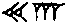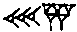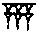# Number of the day

### Number of the day.

Today's number of the day is : 84906 ( eighty-four thousand nine hundred six )

#### Some names to call this number with

• Nominal : 84906
• Cardinal : eighty-four thousand nine hundred six
• Ordinal : 84,906th
• This number as US currency : eighty-four thousand nine hundred six dollars

#### How to write this number in various bases?

• Base 2 (Binary) : 101001011101010102
• Base 3 (Ternary) : 110221102003
• Base 4 (Quaternary) : 1102322224
• Base 5 (Quinary) : 102041115
• Base 6 (Senary) : 14530306
• Base 8 (Octal) : 2456528
• Base 12 (Duodecimal) : 4117612
• Base 16 (Hexadecimal) : 14BAA16
• Base 20 (Vigesimal) : AC5620

#### How to write this number in various other number systems?

• 84906 in Roman Numeral : LXXXMVCMVI
• 84906 in Chinese Numeral : 捌萬肆仟玖佰陸
• 84906 in Egyptian Numeral : 𓂴𓆿𓍪𓏿
• 84906 in Babylonian Numeral :#### Some general facts

• Is 84906 an odd number? : 84906 is NOT an odd number
• Is 84906 an even number? : 84906 is an even number
• Is 84906 a palindrome? : 84906 is NOT a palindrome number
• Is 84906 a triangle number? : 84906 is NOT a triangle number
• Is 84906 a Prime Number? : 84906 is NOT a prime
• Is 84906 a perfect number? : 84906 is NOT a perfect number
• Is 84906 a Mersenne Prime? : 84906 is NOT a Mersenne prime
• Is 84906 a Fermat Prime? : 84906 is NOT a Fermat prime
• Is 84906 a Fibonacci Prime? : 84906 is NOT a Fibonacci prime
• Is 84906 a Partition Prime? : 84906 is NOT a Partition prime
• Is 84906 a Pell Prime? : 84906 is NOT a Pell prime